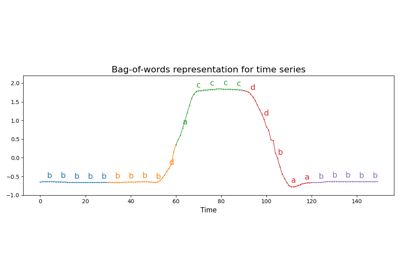# `pyts.bag_of_words`.BagOfWords¶

class `pyts.bag_of_words.``BagOfWords`(window_size=0.5, word_size=0.5, n_bins=4, strategy='normal', numerosity_reduction=True, window_step=1, threshold_std=0.01, norm_mean=True, norm_std=True, overlapping=True, raise_warning=False, alphabet=None)[source]

Bag-of-words representation for time series.

This algorithm uses a sliding window to extract subsequences from the time series and transforms each subsequence into a word using the Piecewise Aggregate Approximation and the Symbolic Aggregate approXimation algorithms. Thus it transforms each time series into a bag of words.

Parameters: window_size : int or float (default = 0.5) Length of the sliding window. If float, it represents a percentage of the size of each time series and must be between 0 and 1. word_size : int or float (default = 0.5) Length of the words. If float, it represents a percentage of the length of the sliding window and must be between 0. and 1. n_bins : int (default = 4) The number of bins to produce. It must be between 2 and `min(n_timestamps, 26)`. strategy : ‘uniform’, ‘quantile’ or ‘normal’ (default = ‘normal’) Strategy used to define the widths of the bins: ‘uniform’: All bins in each sample have identical widths ‘quantile’: All bins in each sample have the same number of points ‘normal’: Bin edges are quantiles from a standard normal distribution numerosity_reduction : bool (default = True) If True, delete sample-wise all but one occurence of back to back identical occurences of the same words. window_step : int or float (default = 1) Step of the sliding window. If float, it represents the percentage of the size of each time series and must be between 0 and 1. The window size will be computed as `ceil(window_step * n_timestamps)`. threshold_std : int, float or None (default = 0.01) Threshold used to determine whether a subsequence is standardized. Subsequences whose standard deviations are lower than this threshold are not standardized. If None, all the subsequences are standardized. norm_mean : bool (default = True) If True, center each subseries before scaling. norm_std : bool (default = True) If True, scale each subseries to unit variance. overlapping : bool (default = True) If True, time points may belong to two bins when decreasing the size of the subsequence with the Piecewise Aggregate Approximation algorithm. If False, each time point belong to one single bin, but the size of the bins may vary. raise_warning : bool (default = False) If True, a warning is raised when the number of bins is smaller for at least one subsequence. In this case, you should consider decreasing the number of bins, using another strategy to compute the bins or removing the corresponding time series. alphabet : None or array-like, shape = (n_bins,) Alphabet to use. If None, the first n_bins letters of the Latin alphabet are used.

References

  J. Lin, R. Khade and Y. Li, “Rotation-invariant similarity in time series using bag-of-patterns representation”. Journal of Intelligent Information Systems, 39 (2), 287-315 (2012).

Examples

```>>> import numpy as np
>>> from pyts.bag_of_words import BagOfWords
>>> X = np.arange(12).reshape(2, 6)
>>> bow = BagOfWords(window_size=4, word_size=4)
>>> bow.transform(X)
array(['abcd', 'abcd'], dtype='<U4')
>>> bow.set_params(numerosity_reduction=False)
BagOfWords(...)
>>> bow.transform(X)
array(['abcd abcd abcd', 'abcd abcd abcd'], dtype='<U14')
```

Methods

 `__init__`([window_size, word_size, n_bins, …]) Initialize self. `fit`(X[, y]) Pass. `fit_transform`(X[, y]) Fit to data, then transform it. `get_params`([deep]) Get parameters for this estimator. `set_params`(**params) Set the parameters of this estimator. `transform`(X) Transform each time series into a bag of words.
`__init__`(window_size=0.5, word_size=0.5, n_bins=4, strategy='normal', numerosity_reduction=True, window_step=1, threshold_std=0.01, norm_mean=True, norm_std=True, overlapping=True, raise_warning=False, alphabet=None)[source]

Initialize self. See help(type(self)) for accurate signature.

`fit`(X, y=None)[source]

Pass.

Parameters: X Ignored y Ignored self : object
`fit_transform`(X, y=None, **fit_params)

Fit to data, then transform it.

Fits transformer to X and y with optional parameters fit_params and returns a transformed version of X.

Parameters: X : array-like of shape (n_samples, n_features) Input samples. y : array-like of shape (n_samples,) or (n_samples, n_outputs), default=None Target values (None for unsupervised transformations). **fit_params : dict Additional fit parameters. X_new : ndarray array of shape (n_samples, n_features_new) Transformed array.
`get_params`(deep=True)

Get parameters for this estimator.

Parameters: deep : bool, default=True If True, will return the parameters for this estimator and contained subobjects that are estimators. params : dict Parameter names mapped to their values.
`set_params`(**params)

Set the parameters of this estimator.

The method works on simple estimators as well as on nested objects (such as `Pipeline`). The latter have parameters of the form `<component>__<parameter>` so that it’s possible to update each component of a nested object.

Parameters: **params : dict Estimator parameters. self : estimator instance Estimator instance.
`transform`(X)[source]

Transform each time series into a bag of words.

Parameters: X : array-like, shape = (n_samples, n_timestamps) Data to transform. X_new : array, shape = (n_samples,) Bags of words.

## Examples using `pyts.bag_of_words.BagOfWords`¶Bag of Words